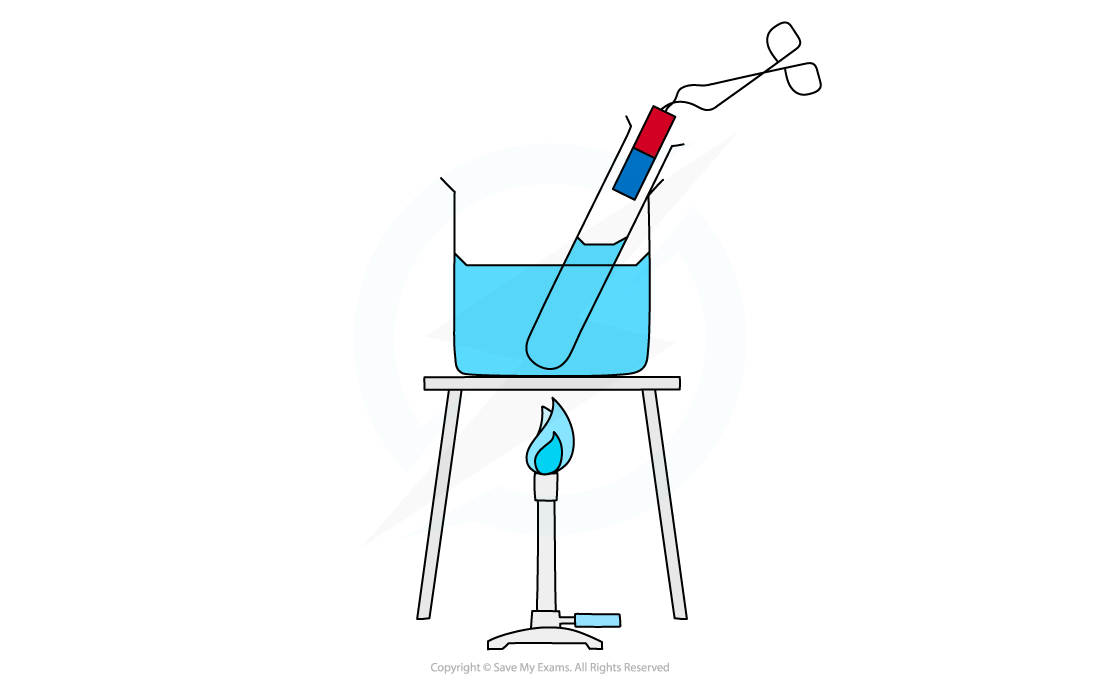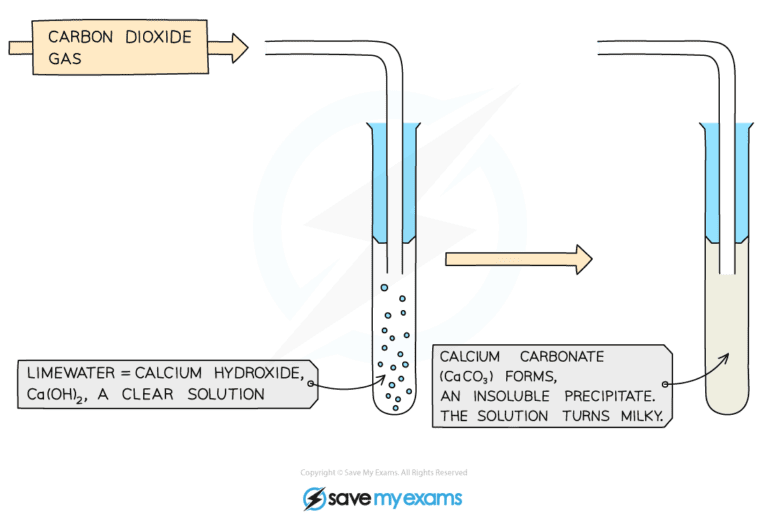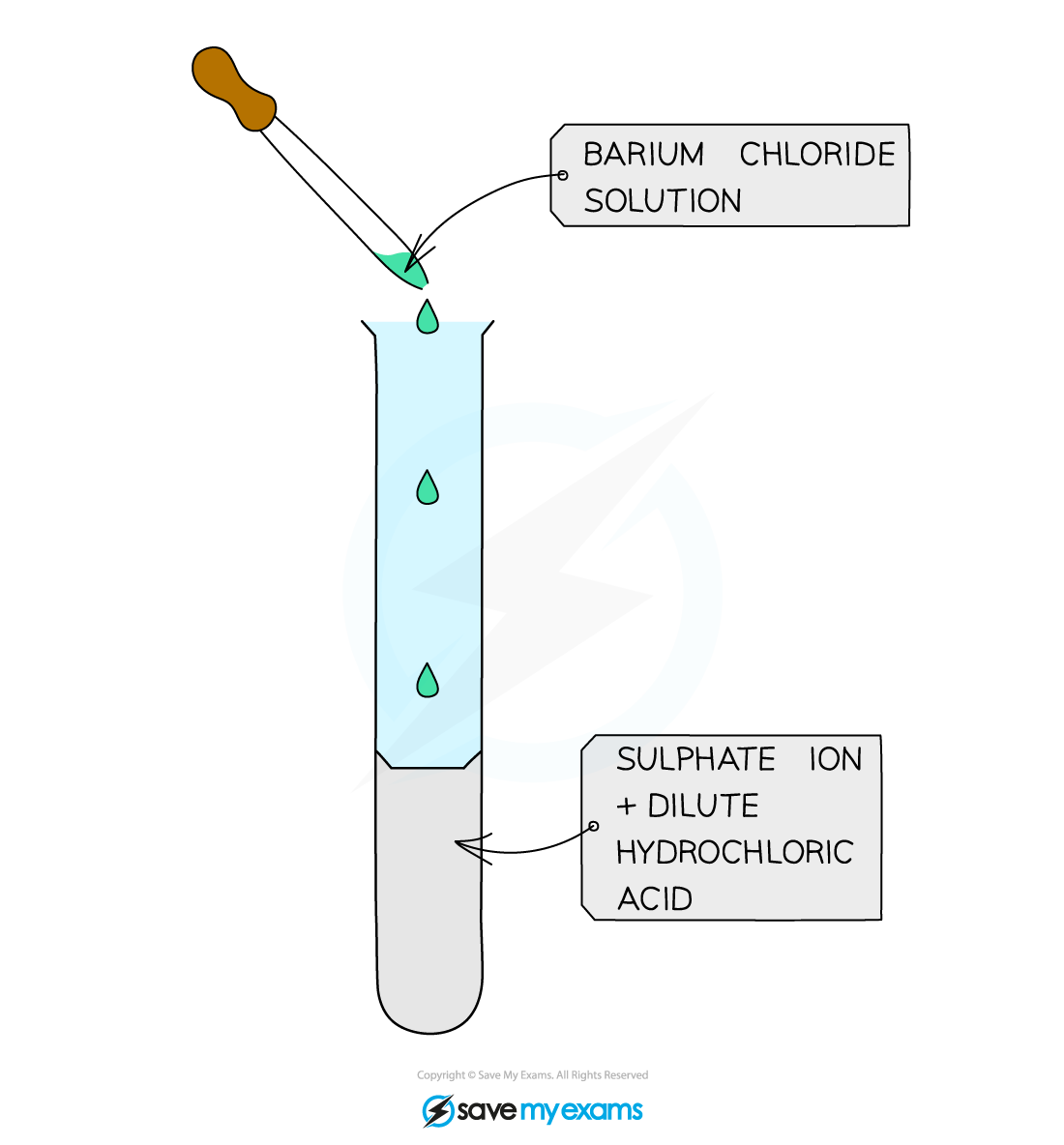# Edexcel A Level Chemistry:复习笔记4.2.4 Qualitative Analysis

### Core Practical 7: Analysis of Inorganic and Organic Unknowns

#### Test Tube Reactions

• Simple test tube reactions can be done to identify the following ions:
• Ammonium ions (NH4+)
• Halide ions (X-) - covered in 2.3.4 Halide Ion Reactions
• Carbonate ions (CO32-) and hydrogencarbonate ions (HCO3-)
• Sulfate ions (SO42-)

• If the sample to be tested is a solid, then it must be dissolved in deionised water and made into an aqueous solution

#### Testing for Ammonium Ions

• About 10 drops of a solution containing ammonium ions, such as ammonium chloride, should be added to a clean test tube
• About 10 drops of sodium hydroxide should be added using a pipette

Overall equation: NH4Cl (aq) + NaOH (aq) → NH3 (g) + H2O (l) + NaCl (aq)

Ionic equation: NH4+ (aq) + OH- (aq) → NH3 (g) + H2O (l)

• The test tube should be swirled carefully to ensure that it is mixed well
• The test tube of the solution should then be placed in a beaker of water, and the beaker of water should be placed above a Bunsen burner, so that it can become a water bath
• As the solution is heated gently, fumes will be produced
• A pair of tongs should be used to hold a damp piece of red litmus paper near the mouth of the test tube, to test the fumes
• The red litmus paper will change colour and become blue in the presence of ammonia gasDamp red litmus paper turning blue in the presence of ammonia gas

#### Testing for Carbonate Ions

• A small amount (around 1 cm3) of dilute hydrochloric acid should be added to a test tube using a pipette
• An equal amount of sodium carbonate solution should then be added to the test tube using a clean pipette

Overall equation: 2HCl (aq) + Na2CO3 (aq) → 2NaCl (aq) + CO2 (g) + H2O (l)

Ionic equation: 2H+ (aq) + CO32- (aq) → CO2 (g) + H2O (l)

• As soon as the sodium carbonate solution is added, a bung with a delivery tube should be attached to the test tube
• The delivery tube should transfer the gas which is formed into a different test tube which contains a small amount of limewater (calcium hydroxide solution)

• Carbonate ions will react with hydrogen ions from the acid to produce carbon dioxide gas
• Carbon dioxide gas will turn the limewater milkyWhen carbon dioxide gas is bubbled into limewater it will turn cloudy as calcium carbonate is produced

• A similar reaction is seen with sodium hydrogen carbonate, but the equations are:

Overall equation: HCl (aq) + NaHCO3 (aq) → NaCl (aq) + CO2 (g) + H2O (l)

Ionic equation: H+ (aq) + HCO3- (aq) → CO2 (g) + H2O (l)

#### Testing for Sulfate Ions

• Acidify the sample with dilute hydrochloric acid and then add a few drops of aqueous barium chloride
• If a sulfate is present then a white precipitate of barium sulfate is formed:

Ba2+ (aq) + SO42- (aq) → BaSO4 (s)A white precipitate of barium sulfate is a positive result for the presence of sulfate ions

#### Exam Tip

HCl is added first to remove any carbonates which may be present and would also produce a precipitate and interfere with the results.